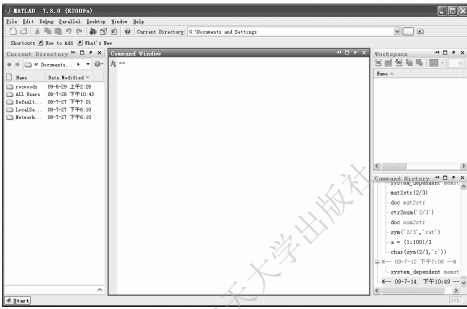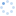# MATLAB高效编程技巧应用与25个案例分析的详细资料概述

### 资料详情

本着让初学者迅速上手的思想，本章由 MATLAB界面入手，介绍基本的 MATLAB操作环境，进而用一系列短小的程序帮助读者建立起对 MATLAB的最初印象，激发学习兴趣。在这之后对 M 语言进行简明扼要介绍以帮助读者能自己编写 MATLAB程序。最后一节是笔者长期使用、学习 MATLAB并在国内众多 MATLAB 论坛与各层次 MATLAB 使用者交流的经验总结，相信无论读者以前用没用过 MATLAB，都能从中得到收获

Windows环境下，MATLAB常用启动方法有以下几种：

1）双击桌面上的 MATLAB 快捷方式图标（一般指向 MATLAB 安装目录下 bin文件 matlab.exe文件）;

2）依次单击 Windows任务栏上“开始”按钮→“所有程序”→MATLAB，找到 MATLAB R2009a选项（其他版本找到对应选项）进入 MATLAB界面;

3）单击 Windows任务栏上“开始”按钮，在弹出的开始菜单中单击“运 行”按 钮，输 入 MATLAB并按 Enter键进入 MATLAB界面。

第一次启动 MATLAB完毕后，呈现给读者的是如图1.1所示的 MATLAB界面（也即默认状态下的桌面）。对于初学者来说，最主要需要了解以下三个窗口，即命令窗口（Command Window）、工作空间（Workspace）和历史命令（CommandHistory）窗口。

命令窗口是用来输入各种 MATLAB命令，观察运算结果的窗口。

工作空间可以用来浏览程序运行过程中或完毕后得到的变量的值，当工作空间有变量后， 可以选中要观察的变量，双击它，MATLAB会新开一个窗口来展示这个变量的值或者属性。

历史命令窗口里面记录了曾经在 Command Window 中输入过的命令。当选中的是历史命令窗口或者命令窗口时，可以按键盘上的上、下方向键来选中或者调出上一条、下一条指令。双击历史命令窗口中某条指令，则会在命令窗口中重新运行这条指令。

由于本书的侧重点不在基础知识上，限于篇幅，关于这些窗口的详细介绍就略去了。需要说明的是，MATLAB 这些窗口操作非常人性化，人机交互性非常好。读者如果初次使 用 MATLAB，可以按照自己的 Windows系统使用经验来试探着操作这些窗口、按钮，观察结果，很快就会熟悉 MATLAB基本的操作环境。如果对某些图标的意义不清楚，可以把鼠标指针分别移到相应的图标上面（不要点击），过一两秒钟，就会显示当前图标的功能介绍。如果在操作过程中关闭了某些窗口，譬如命令窗口、工作空间等而改变了桌面结构，想要恢复默认桌面显示状态，只需单击菜单栏里Desktop菜单项，进入后单击DesktopLayout，选择Default就恢复默认桌面结构了。### 相关资源

elecfans网友上传我的资料

最新分享

最新评论